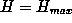Next: Acknowledgement: Up: Appendices Previous: Appendices

## A: Pseudo-Code of FAMUSAMM

In this section we provide a summarizing pseudo-code description of FAMUSAMM. For clarity, we have omitted the special algorithmic steps, which are necessary during the start-up phase or are associated with hierarchical grouping and recalculation of the interaction list. As to decide, whether in a given time step forces or local Taylor expansions have to be explicitly calculated or may be extrapolated, we introduce a variable M, which denotes the highest hierarchy level H of SAMM for which an explicit calculation has to be performed (cf. Figure 4). M is called extrapolation level. The following abbreviations are used: LTE: local Taylor expansion, MM: multipole moments, SU: structural unit. Indentation defines the grouping of instructions.

```
For every integration step:

Set extrapolation level M.

Set all LTE coefficients to zero.

Calculate MMs of all SUs (hierarchy level H=1).

For H=2 to:

Calculate MMs for each cluster on higher hierarchy levels H.

Fordown to H=1:

For every object at level H:

If H>M:

Extrapolate LTE of hierarchy level H.

Else:

Sum up contributions to LTE at level H according to interaction list

If H>1:

Inherit LTE of level H to level H-1.

For every atom:

Compute non-electrostatic forces forces (van der Waals, chem. binding forces).

Add Coulomb forces from LTE of SU.

Add Coulomb forces from atoms in distance class j=0 (cf. Figure 4).

If integration step even:

Add calculated forces from distance class j=1.

Else:

Add extrapolated forces from distance class j=1.

Perform Verlet integration step.

```

Helmut Grubmueller
Wed Apr 30 15:40:09 MET DST 1997